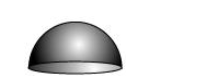# Find the total surface area of a hemisphere of radius 10 cm.Question. Find the total surface area of a hemisphere of radius $10 \mathrm{~cm}$. [Use $\pi=3.14$ ]

Solution:Radius (r) of hemisphere = 10 cm

Total surface area of hemisphere = CSA of hemisphere + Area of circular end of hemisphere

$=2 \pi r^{2}+\pi r^{2}$

$=3 \pi r^{2}$

$=\left[3 \times 3.14 \times(10)^{2}\right] \mathrm{cm}^{2}$

$=942 \mathrm{~cm}^{2}$

Therefore, the total surface area of such a hemisphere is $942 \mathrm{~cm}^{2}$.